# 前言

• 创建一个预制体（prefab
• 实例化（Instantiate）多个立方体
• 图形化一个数学函数
• 将图形赋予动画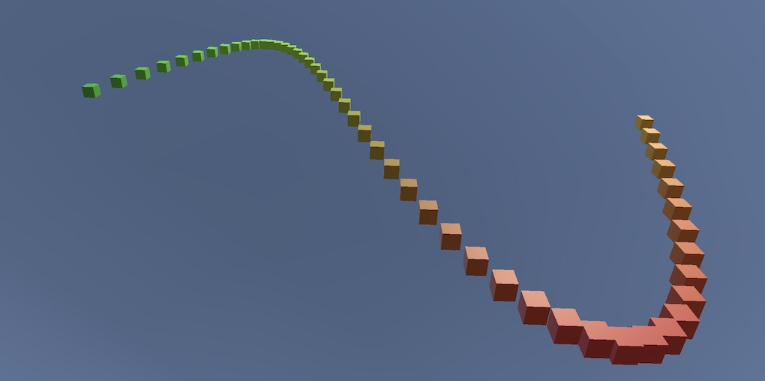# 创建一排"点"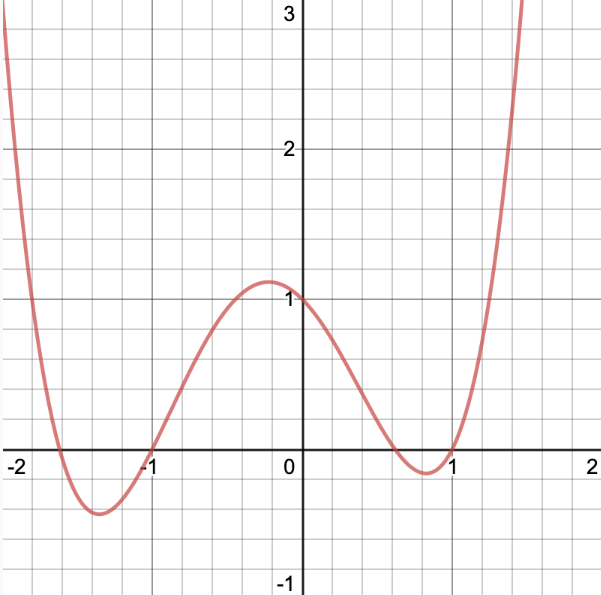## 创建预制体(Prefabs)

你也可以使用粒子系统或者线段，但是立方体是最容易使用的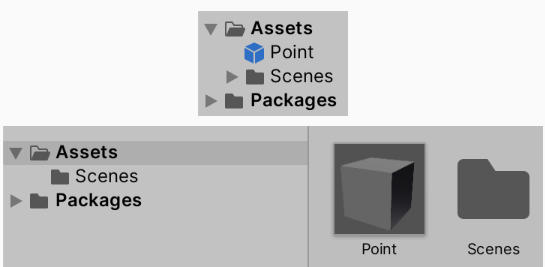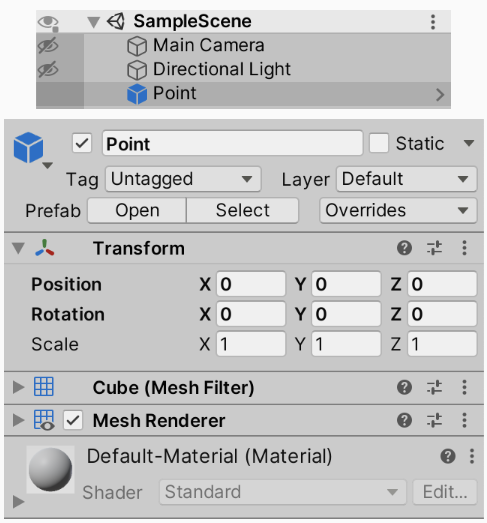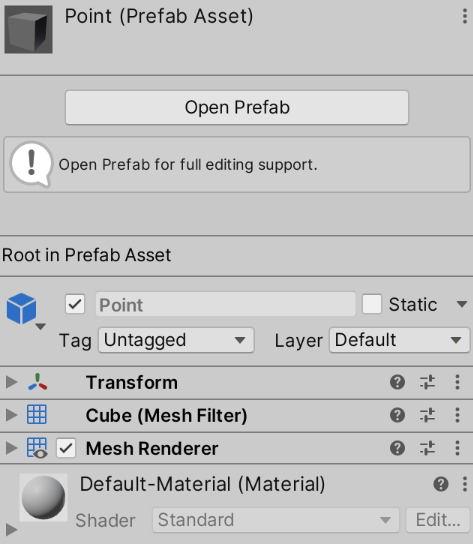如果你是通过项目资源面板（Project）的预制体进入的预制体场景，则其默认是关闭天空盒（Skybox）的。



## 创建图形组件(类)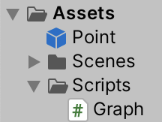using UnityEngine;

public class Graph : MonoBehaviour
{
[SerializeField]
Transform pointPrefab;  //序列化 Transform 类型的字段
}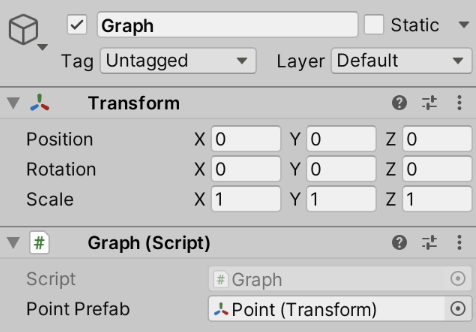## 实例化预制体

using UnityEngine;

public class Graph : MonoBehaviour
{
[SerializeField]
Transform pointPrefab;  //序列化 Transform 类型的字段

void Awake()
{
Object.Instantiate(pointPrefab);	//实例化预制体
}
}


MonoBehaviour 继承自 Behaviour，而 Behaviour 继承自 Component
Component 继承自 Object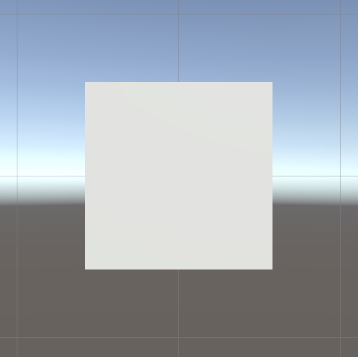void Awake()
{
Transform point = Instantiate(pointPrefab);
}


void Awake()
{
Transform point = Instantiate(pointPrefab);
point.localPosition = Vector3.right;
}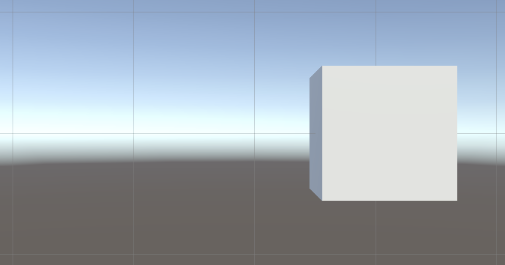void Awake()
{
Transform point = Instantiate(pointPrefab);
point.localPosition = Vector3.right;

Transform point = Instantiate(pointPrefab);
point.localPosition = Vector3.right*2f;
}


void Awake()
{
Transform point = Instantiate(pointPrefab);
point.localPosition = Vector3.right;

point = Instantiate(pointPrefab);
point.localPosition = Vector3.right*2f;
}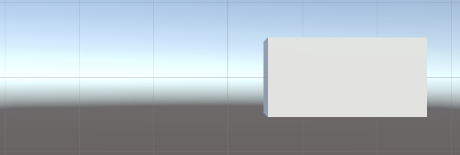## 循环创建

void Awake()
{
while ()
{
Transform point = Instantiate(pointPrefab);
point.localPosition = Vector3.right;
}
}


while关键词必须后面跟着圆括号内的表达式。只有圆括号内的表达式计算结果为真（True）时，才会执行后面代码块的内容。之后，程序会再次回到while语句，再次判断圆括号内的表达式结果，如果为真，则继续执行，如此重复。但是需要注意的是，要确保循环不会永远重复，即不会产生无限循环导致程序卡住，最安全的编译是使用关键词false，代码示例：

while (false)
{
Transform point = Instantiate(pointPrefab);
point.localPosition = Vector3.right;
}


void Awake()
{
int i = 0;
while (i<10)
{
i = i + 1;
Transform point = Instantiate(pointPrefab);
point.localPosition = Vector3.right;
}
}


point.localPosition = Vector3.right * i;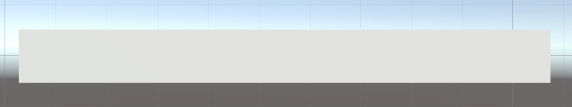int i = 0;
while (i<10)
{
Transform point = Instantiate(pointPrefab);
point.localPosition = Vector3.right*i;
i = i + 1;
}


## 简化代码

void Awake()
{
for (int i = 0; i < 10; i++){
Transform point = Instantiate(pointPrefab);
point.localPosition = Vector3.right*i;
}
}


## 更改实例化范围

for (int i = 0; i < 10; i++){
Transform point = Instantiate(pointPrefab);
point.localPosition = Vector3.right*i;
point.localScale = Vector3.one / 5f;	//缩小5分之1
}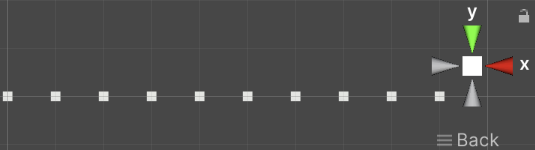for (int i = 0; i < 10; i++){
Transform point = Instantiate(pointPrefab);
point.localPosition = Vector3.right*i / 5f;	//位置也除以5
point.localScale = Vector3.one / 5f;
}


point.localPosition = Vector3.right* (i / 5f -1f);


point.localPosition = Vector3.right* (i + 0.5f / 5f -1f);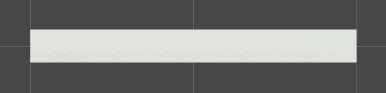## 优化代码结构

var scale = Vector3.one / 5f;	//提到外面，仅计算一次即可
for (int i = 0; i < 10; i++){
Transform point = Instantiate(pointPrefab);
point.localPosition = Vector3.right* ((i + 0.5f) / 5f -1f);
point.localScale = scale;
}


Vector3 position;   //位置变量
var scale = Vector3.one / 5f;
for (int i = 0; i < 10; i++){
Transform point = Instantiate(pointPrefab);
position.x = ((i + 0.5f) / 5f - 1f);    //计算点的 X 轴的位置
point.localPosition = position; //将实例物体位置调整到计算好的位置
point.localScale = scale;
}


Vector3 类型结构有三个浮点类型字段：x，y和z。这些字段是公开的（Public），因此我们可以单独更改它们。


var position = Vector3.zero;   //位置变量
var scale = Vector3.one / 5f;
for (int i = 0; i < 10; i++){
Transform point = Instantiate(pointPrefab);
position.x = ((i + 0.5f) / 5f - 1f);    //计算点的 X 轴的位置
point.localPosition = position; //将实例物体位置调整到计算好的位置
point.localScale = scale;
}


## 使用 X 映射 Y 的值

var position = Vector3.zero;   //位置变量
var scale = Vector3.one / 5f;
for (int i = 0; i < 10; i++){
Transform point = Instantiate(pointPrefab);
position.x = ((i + 0.5f) / 5f - 1f);    //计算点的 X 轴的位置

position.y = position.x;    //Y 轴的值由 X 的值映射

point.localPosition = position; //将实例物体位置调整到计算好的位置
point.localScale = scale;
}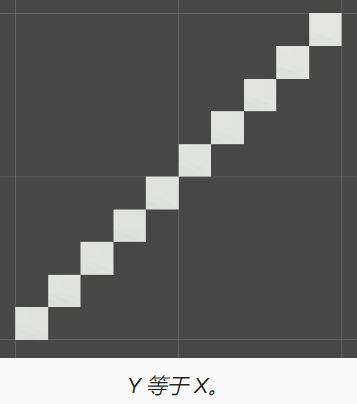position.y = position.x*position.x;    //Y 轴新的映射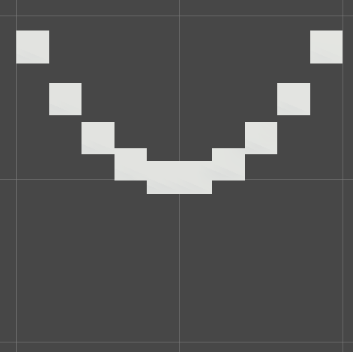# 创建更多的"点"

## 动态修改"点"数

[SerializeField]
int resolution = 10;    //立方体的数量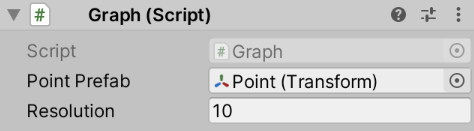[SerializeField,Range(10,100)]
int resolution = 10;    //立方体的数量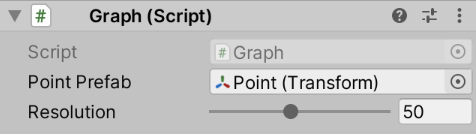Range属性仅仅检查我们在检查器面板（Inspector）的改动，它不会影响其以外的修改。



## 实例化变量

for (int i = 0; i < resolution; i++) {
//省略中间代码
}


var position = Vector3.zero;   //位置变量
var step = 2f / resolution; //计算应该怎么分配位置
var scale = Vector3.one * step;
for (int i = 0; i < resolution; i++){
Transform point = Instantiate(pointPrefab);
position.x = ((i + 0.5f) * step - 1f);    //计算点的 X 轴的位置

position.y = position.x*position.x;    //Y 轴的值由 X 的值映射

point.localPosition = position; //将实例物体位置调整到计算好的位置
point.localScale = scale;
}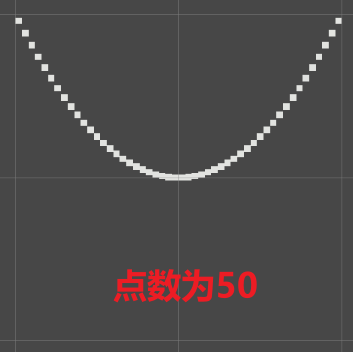## 设置实例物体父对象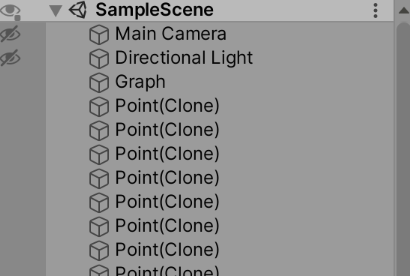float step = 2f / resolution; //计算应该怎么分配位置
var position = Vector3.zero;   //位置变量
var scale = Vector3.one * step;
for (int i = 0; i < resolution; i++){
Transform point = Instantiate(pointPrefab);
position.x = (i + 0.5f) * step - 1f;    //计算点的 X 轴的位置
position.y = position.x*position.x;    //Y 轴的值由 X 的值映射
point.localPosition = position; //将实例物体位置调整到计算好的位置
point.localScale = scale;
point.SetParent(transform);	//设置父对象
}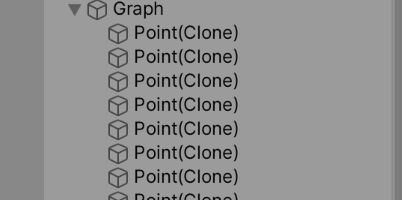point.SetParent(transform,false);


# 为图形添加颜色

GPU 运行着色器（Shader）程序来渲染 3D 对象。Unity 材质资源确定使用哪个着色器，并允许配置其属性。我们需要通过 Assets/Create/Shader/Standard Surface Shader 创建一个自定义着色器并命名为Point Surface来实现我们想要的功能。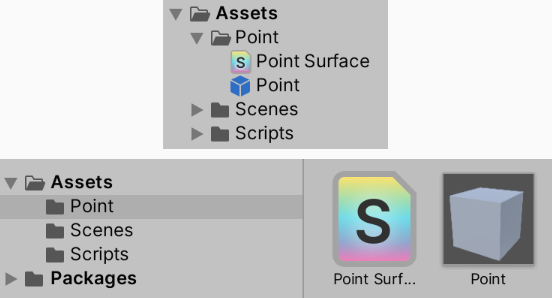Unity 提供了一个框架来快速生成执行默认光照计算的着色器，你可以通过修改它的某些值来影响它的结果。



Unity 有着自己的着色器语法，总体上大致类似于C#，但是它是不同语言的混合。它以关键字Shader作为开头，后面跟着定义着色器在菜单栏显示的名称字符串。字符串写在双引号内。我们使用字符串 “Graph/Point Surface”，最后以花括号结束，花括号其中包含着色器内容代码。

Shader "Graph/Point Surface"{}


Shader "Graph/Point Surface"{
}


Shader "Graph/Point Surface"{
FallBack "DIFFUSE"
}


Shader "Graph/Point Surface"{
CGPROGRAM

ENDCG
}
FallBack "DIFFUSE"
}


Shader "Graph/Point Surface"{
CGPROGRAM
ENDCG
}
FallBack "DIFFUSE"
}


pragma是什么意思？

pragma 这个词来自于希腊语，指的是一个动作，或者是需要做的事情



Shader "Graph/Point Surface"{
CGPROGRAM
#pragma target 3.0
ENDCG
}
FallBack "DIFFUSE"
}


Shader "Graph/Point Surface"{
CGPROGRAM
#pragma target 3.0

struct Input {
float3 worldPos;
};
ENDCG
}
FallBack "DIFFUSE"
}


是的，使用这种方法，只要物体离开 Graph 物体的位置就会变化



struct Input {
float3 worldPos;
};

void ConfigureSurface(Input input,SurfaceOutputStandard surface){}


void ConfigureSurface(Input input,inout SurfaceOutputStandard surface){}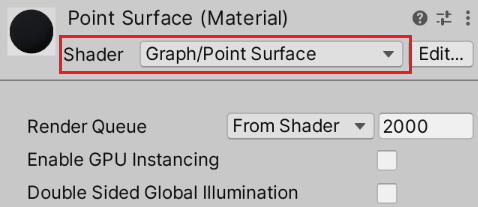void ConfigureSurface(Input input,inout SurfaceOutputStandard  surface){
surface.Smoothness = 0.5;
}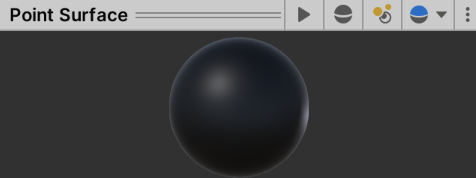float _Smoothness;

void ConfigureSurface(Input input,inout SurfaceOutputStandard  surface){
surface.Smoothness = _Smoothness;
}


Shader "Graph/Point Surface"{
Properties{
_Smoothness ("Smoothness",Range(0,1)) = 0.5
}

CGPROGRAM
#pragma target 3.0

struct Input {
float3 worldPos;
};

float _Smoothness;

void ConfigureSurface(Input input,inout SurfaceOutputStandard  surface){
surface.Smoothness = _Smoothness;
}

ENDCG
}
FallBack "DIFFUSE"
}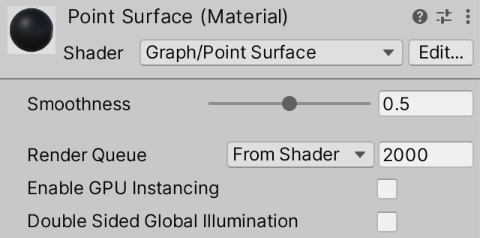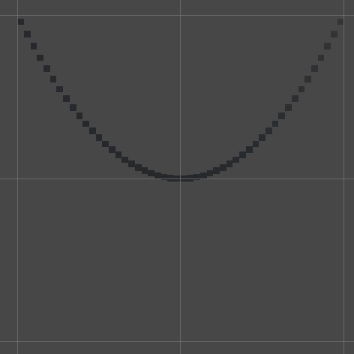## 基于世界坐标开始着色

void ConfigureSurface(Input input,inout SurfaceOutputStandard  surface){
surface.Albedo = input.worldPos;
surface.Smoothness = _Smoothness;
}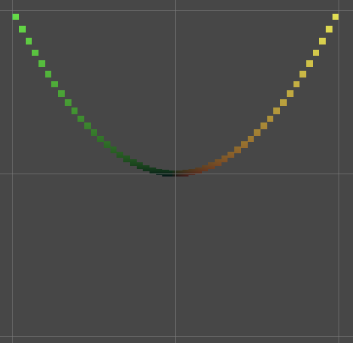Albedo（漫反射）在拉丁语中是白色的意思。它是衡量有多少光被表面漫反射的度量。



surface.Albedo = input.worldPos* 0.5 +0.5;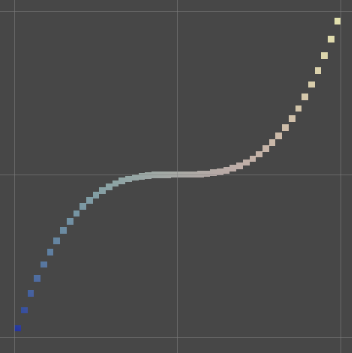surface.Albedo.rg = input.worldPos.xy* 0.5 +0.5;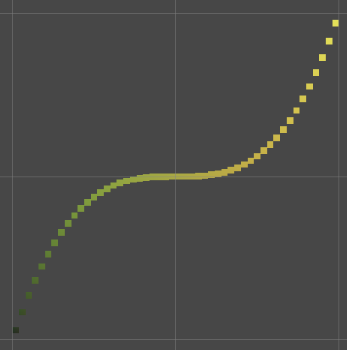## 通用渲染线管（Universal Render Pipeline）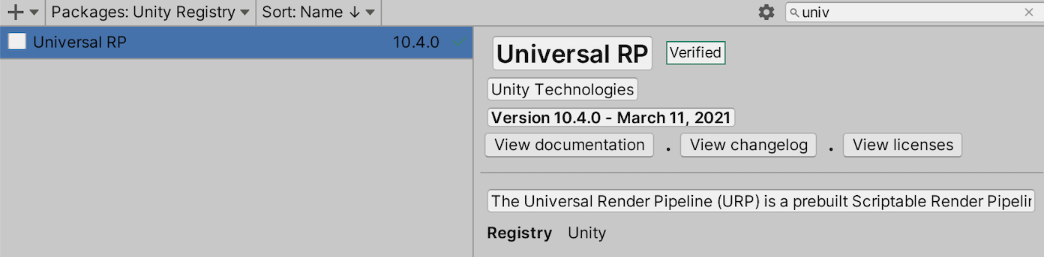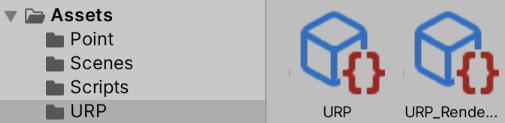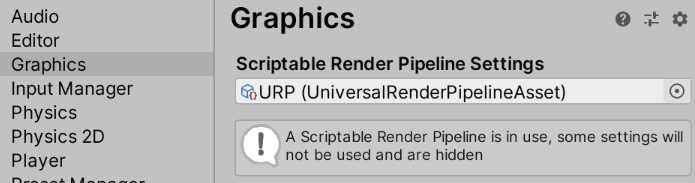HDRP 呢？

HDRP 是一个更复杂的渲染管线，我并不会我的教程中介绍它。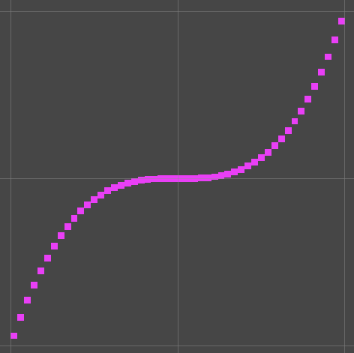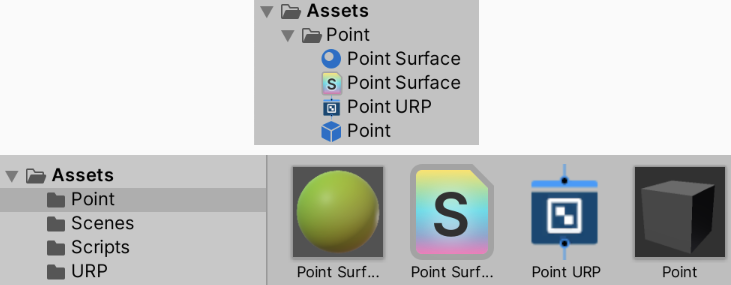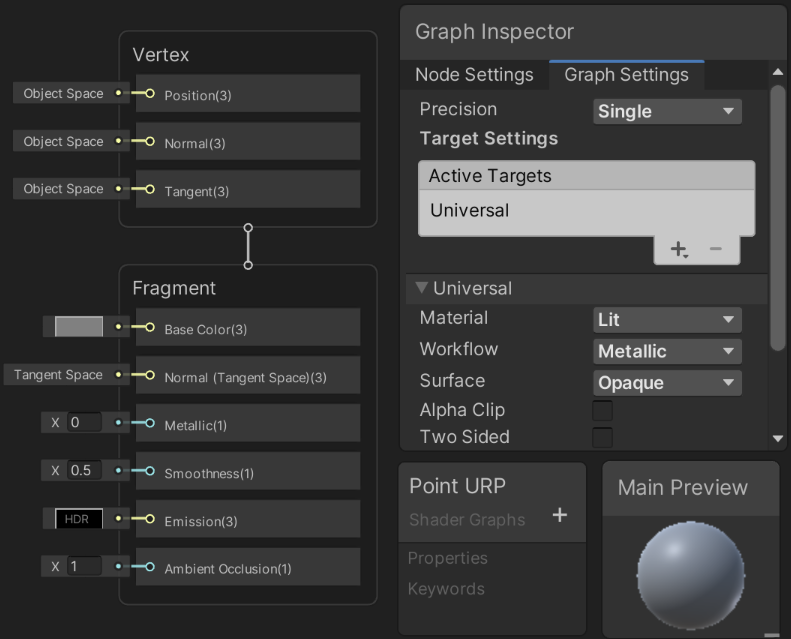URP 图形化着色器由表示数据或者操作的节点组成。现在"面"（Fragment）节点的Smoothness值为 0.5 。要使其成为可配置的着色器属性，需要在左边的 Ponit URP “黑板”（Blackboard）点击加号按钮，选择Float类型，并将其命名为Smoothness（这个过程是添加变量）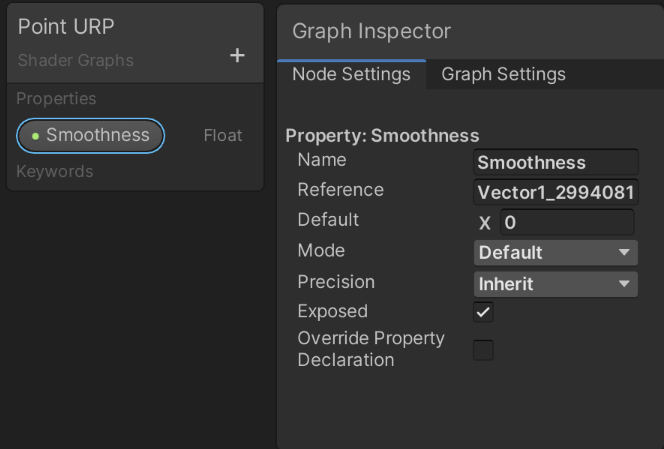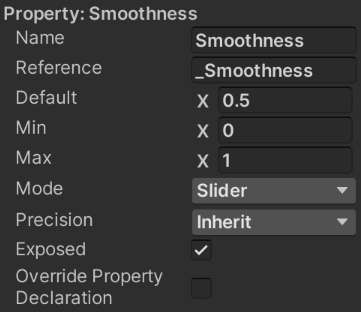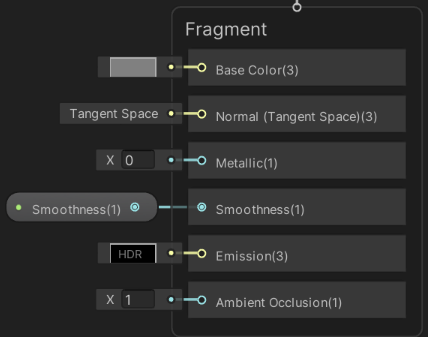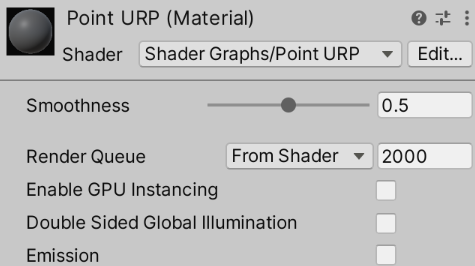## 使用节点编程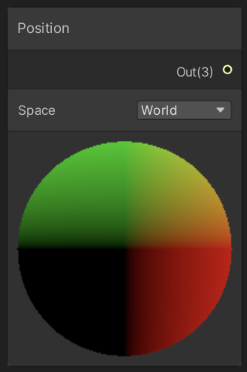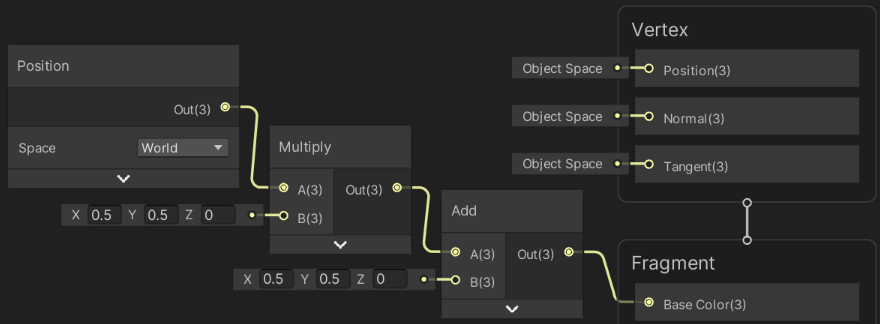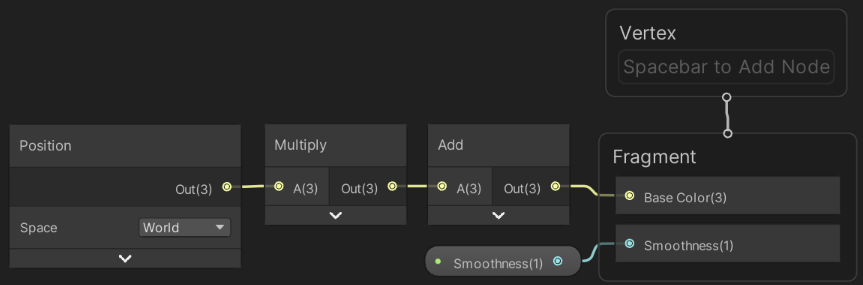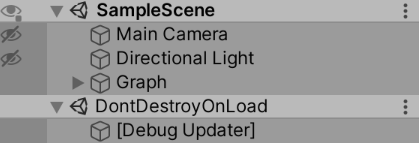# 为图形添加动画

## 获取“点”引用

[SerializeField,Range(10,100)]
int resolution = 50;    //立方体的数量

Transform points;


Transform[] points;


points字段现在是对数组的引用，其元素类型为 Transform。数组是对象，而不是简单的值。我们必须显式的创建一个这样的对象并让字段获取对其的引用。这是通过关键字new来实现的。

void Awake(){
points = new Transform[];
}


points = new Transform[resolution];


points = new Transform[resolution]; //创建数组对象
for (int i = 0; i < resolution; i++)
{
Transform point = Instantiate(pointPrefab); //获取实例化对象的引用
points[i] = point;  //填充数组
}


points = new Transform[resolution]; //创建数组对象
for (int i = 0; i < resolution; i++)
{
Transform point = points[i] =  Instantiate(pointPrefab); //获取实例化对象的引用并填充数组
}


for (int i = 0; i < points.Length; i++)
{
Transform point = points[i] =  Instantiate(pointPrefab); //获取实例化对象的引用并填充数组
}


## 更新“点”

//这句不需要了
position.y = position.x*position.x* position.x;    //Y 轴的值由 X 的值映射


Awake一样在Updat添加一个带有for循环的代码，但是它的块中代码还暂时是空的。

void Update()
{
for (int i = 0; i < points.Length; i++)
{
//这里啥也没有
}
}


void Update()
{
for (int i = 0; i < points.Length; i++)
{
Transform point = points[i];
}
}


for (int i = 0; i < points.Length; i++)
{
Transform point = points[i];
Vector3 position = point.localPosition;
}


Transform point = points[i];
Vector3 position = point.localPosition;
position.y = position.x * position.x * position.x;


Transform point = points[i];    //获取实例对象
Vector3 position = point.localPosition; //获取对象的位置
position.y = position.x * position.x * position.x;  //计算新的位置
point.localPosition = position; //新位置赋值给对象


## 显示正弦图形

position.y = Mathf.Sin(position.x);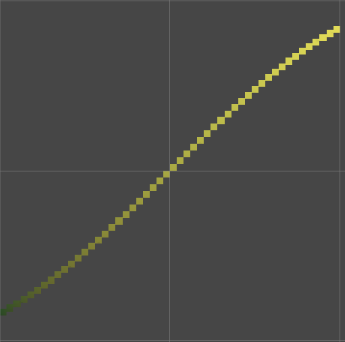它是 UnityEngine 命名空间中一个结构，包含了数学函数和常量的集合。



position.y = Mathf.Sin(Mathf.PI * position.x);  //计算新的位置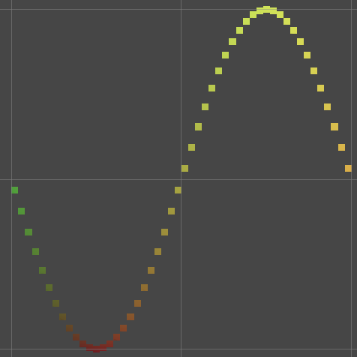position.y = Mathf.Sin(Mathf.PI * (position.x + Time.time));  //计算新的位置


float time = Time.time; //获取时间
for (int i = 0; i < points.Length; i++)
{
Transform point = points[i];    //获取实例对象
Vector3 position = point.localPosition; //获取对象的位置
position.y = Mathf.Sin(Mathf.PI * (position.x + time));  //计算新的位置
point.localPosition = position; //新位置赋值给对象
}


## 限制颜色范围

surface.Albedo.rg = saturate(input.worldPos.xy * 0.5 + 0.5);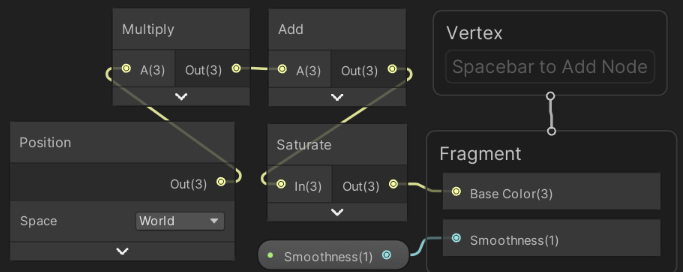# End

Q.E.D.

••